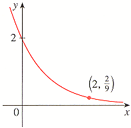# Find the exponential function f(x) = cax whose graph is given.

Find the exponential function f(x) =
Cax whose graph is given. f x Cax x f(x) = f xx=0,f(x)=2
2=ca^0=c*1=c =>c=2
at x=2, f(x)=2/9
therfore 2/9=2a^2 =>a=1/3
f(x)=2(1/3)^x